# Chapter 2 Introduction to R

This Exploratory Data Analysis section lays the foundation for exploratory data analysis using the R language and packages especially within the tidyverse. This foundation progresses through:

• Introduction : An introduction to the R language
• Abstraction 3 : Exploration of data via reorganization using dplyr 3.4 and other packages in the tidyverse 3.1
• Visualization 4 : Adding visual tools to enhance our data exploration
• Transformation 5 : Reorganizing our data with pivots 5.4 and data joins 5.1In this chapter we’ll introduce the R language, using RStudio to explore its basic data types, structures, functions and programming methods in base R. We’re assuming you’re either new to R or need a refresher. Later chapters will add packages that extend what you can do with base R for data abstraction, transformation, and visualization, then explore the spatial world, statistical models and time series applied to environmental research.

The following code illustrates a few of the methods we’ll explore in this chapter:

temp <- c(10.7, 9.7, 7.7, 9.2, 7.3)
elev <- c(52, 394, 510, 564, 725)
lat <- c(39.52, 38.91, 37.97, 38.70, 39.09)
elevft <- round(elev / 0.3048)
deg <- as.integer(lat)
min <- as.integer((lat-deg) * 60)
sec <- round((lat-deg-min/60)*3600)
sierradata <- cbind(temp, elev, elevft, lat, deg, min, sec)
mydata
##   temp elev elevft   lat deg min sec
## 1 10.7   52    171 39.52  39  31  12
## 2  9.7  394   1293 38.91  38  54  36
## 3  7.7  510   1673 37.97  37  58  12
## 4  9.2  564   1850 38.70  38  42   0
## 5  7.3  725   2379 39.09  39   5  24

RStudio

If you’re new to RStudio, or would like to learn more about using it, there are plenty of resources you can access to learn more about using it. As with many of the major packages we’ll explore, there’s even a cheat sheet: https://www.rstudio.com/resources/cheatsheets/. Have a look at this cheat sheet while you have RStudio running, and use it to learn about some of its different components:

• The Console where you’ll enter short lines of code, install packages, and get help on functions. Messages created from running code will also be displayed here. There are other tabs in this area (e.g. Terminal, R Markdown) we may explore a bit, but mostly we’ll use the console.
• The Source Editor where you’ll write full R scripts and R Markdown documents. You should get used to writing complete scripts and R Markdown documents as we go through the book.
• Various Tab Panes such as the Environment pane where you can explore what scalars and more complex objects contain.
• The Plots pane in the lower right for static plots (graphs & maps that aren’t interactive), which also lets you see a listing of Files, or View interactive maps and maps.

## 2.1 Data Objects

As with all programming 2.8 languages, R works with data and since it’s an object-oriented language, these are data objects. Data objects can range from the most basic type – the scalar which holds one value, like a number or text – to everything from an array of values to spatial data for mapping or a time series of data.

### 2.1.1 Scalars and Assignment

We’ll be looking at a variety of types of data objects, but scalars are the most basic type, holding individual values, so we’ll start with it. Every computer language, like in math, stores values by assigning them constants or results of expressions. These are often called “variables” but we’ll be using that name to refer to a column of data stored in a data frame, which we’ll look at later in this chapter. R uses a lot of objects, and not all are data objects; we’ll also create functions 2.8.1, a type of object that does something (runs the function code you’ve defined for it) with what you provide it.

To create a scalar (or other data object), we’ll use the most common type of statement, the assignment statement, that takes an expression and assigns it to a new data object that we’ll name. The class of that data object is determined by the class of the expression provided, and that expression might be something as simple as a constant like a number or a character string of text. Here’s an example of a very basic assignment statement that assigns the value of a constant 5 to a new scalar x:

x <- 5

Note that this uses the assignment operator <- that is standard for R. You can also use = as most languages do (and I sometimes do), but we’ll use = for other types of assignments.

All object names must start with a letter, have no spaces, and not use any names that are built into the R language or used in package libraries, such as reserved words like for or function names like log. Object names are case-sensitive (which you’ll probably discover at some point by typing in something wrong and getting an error).

x <- 5
y <- 8
Longitude <- -122.4
Latitude <- 37.8
my_name <- "Inigo Montoya"

To check the value of a data object, you can just enter the name in the console, or even in a script or code chunk.

x
##  5
y
##  8
Longitude
##  -122.4
Latitude
##  37.8
my_name
##  "Inigo Montoya"

This is counter to the way printing out values commonly works in other programming languages, and you will need to know how this method works as well because you will want to use your code to develop tools that accomplish things, and there are also limitations to what you can see by just naming objects.

To see the values of objects in programming mode, you can also use the print() function (but we rarely do); or to concatenate character string output, use paste() or paste0.

print(x)
paste0("My name is ", my_name, ". You killed my father. Prepare to die.")

Numbers concatenated with character strings are converted to characters.

paste0(paste("The Ultimate Answer to Life", "The Universe",
"and Everything is ... ", sep=", "),42,"!")
paste("The location is latitude", Latitude, "longitude", Longitude)
##  "The location is latitude 37.8 longitude -122.4"

Review the code above and what it produces. Without looking it up, what’s the difference between paste() and paste0()?

We’ll use paste0() a lot in this book to deal with long file path which create problems for the printed/pdf version of this book, basically extending into the margins. Breaking the path into multiple strings and then combining them with paste0() is one way to handle them. For instance, in the Imagery and Classification Models chapter, the Sentinel2 imagery is provided in a very long file path. So here’s how we use paste0() to recombine after breaking up the path, and we then take it one more step and build out the full path to the 20 m imagery subset.

imgFolder <- paste0("S2A_MSIL2A_20210628T184921_N0300_R113_T10TGK_20210628T230915.",
"SAFE/GRANULE/L2A_T10TGK_A031427_20210628T185628")
img20mFolder <- paste0("~/sentinel2/",imgFolder,"/IMG_DATA/R20m")

## 2.2 Functions

Just as in regular mathematics, R makes a lot of use of functions that accept an input and create an output:

log10(100)
log(exp(5))
cos(pi)
sin(90 * pi/180)

But functions can be much more than numerical ones, and R functions can return a lot of different data objects. You’ll find that most of your work will involve functions, from those in base R to a wide variety in packages you’ll be adding. You will likely have already used the install.packages() and library() functions that add in an array of other functions.

Later in this chapter, we’ll also learn how to write our own functions, a capability that is easy to accomplish and also gives you a sense of what developing your own package might be like.

Arithmetic operators There are of course all the normal arithmetic operators (that are actually functions) like plus + and minus - or the key-stroke approximations of multiply * and divide / operators. You’re probably familiar with these approximations from using equations in Excel if not in some other programming language you may have learned. These operators look a bit different from how they’d look when creating a nicely formatted equation.

For example, $$\frac{NIR - R}{NIR + R}$$ instead has to look like (NIR-R)/(NIR+R).

Similarly * must be used to multiply; there’s no implied multiplication that we expect in a math equation like $$x(2+y)$$ which would need to be written x*(2+y).

In contrast to those four well-known operators, the symbol used to exponentiate – raise to a power – varies among programming languages. R uses either ** or ^ so the the Pythagorean theorem $$c^2=a^2+b^2$$ might be written c**2 = a**2 + b**2 or c^2 = a^2 + b^2 except for the fact that it wouldn’t make sense as a statement to R. Why?

And how would you write an R statement that assigns the variable c an expression derived from the Pythagorean theorem? (And don’t use any new functions from a Google search – from deep math memory, how do you do $$\sqrt{x}$$ using an exponent?)

It’s time to talk more about expressions and statements.

## 2.3 Expressions and Statements

The concepts of expressions and statements are very important to understand in any programming language.

An expression in R (or any programming language) has a value just like an object has a value. An expression will commonly combine data objects and functions to be evaluated to derive the value of the expression. Here are some examples of expressions:

5
x
x*2
sin(x)
(a^2 + b^2)^0.5
(-b+sqrt(b**2-4*a*c))/2*a
paste("My name is", aname)

Note that some of those expressions used previously assigned objects – x, a, b, c, aname.

An expression can be entered in the console to display its current value, and this is commonly done in R for objects of many types and complexity.

cos(pi)
##  -1
Nile
## Time Series:
## Start = 1871
## End = 1970
## Frequency = 1
##    1120 1160  963 1210 1160 1160  813 1230 1370 1140  995  935 1110  994 1020
##    960 1180  799  958 1140 1100 1210 1150 1250 1260 1220 1030 1100  774  840
##    874  694  940  833  701  916  692 1020 1050  969  831  726  456  824  702
##   1120 1100  832  764  821  768  845  864  862  698  845  744  796 1040  759
##    781  865  845  944  984  897  822 1010  771  676  649  846  812  742  801
##   1040  860  874  848  890  744  749  838 1050  918  986  797  923  975  815
##   1020  906  901 1170  912  746  919  718  714  740

Whoa, what was that? We entered the expression Nile and got a bunch of stuff! Nile is a type of data object called a time series that we’ll be looking at much later, and since it’s in the built-in data in base R, just entering its name will display it. And since time series are also vectors which are like entire columns, rows or variables of data, we can vectorize it (apply mathematical operations and functions element-wise) in an expression:

Nile * 2
## Time Series:
## Start = 1871
## End = 1970
## Frequency = 1
##    2240 2320 1926 2420 2320 2320 1626 2460 2740 2280 1990 1870 2220 1988 2040
##   1920 2360 1598 1916 2280 2200 2420 2300 2500 2520 2440 2060 2200 1548 1680
##   1748 1388 1880 1666 1402 1832 1384 2040 2100 1938 1662 1452  912 1648 1404
##   2240 2200 1664 1528 1642 1536 1690 1728 1724 1396 1690 1488 1592 2080 1518
##   1562 1730 1690 1888 1968 1794 1644 2020 1542 1352 1298 1692 1624 1484 1602
##   2080 1720 1748 1696 1780 1488 1498 1676 2100 1836 1972 1594 1846 1950 1630
##   2040 1812 1802 2340 1824 1492 1838 1436 1428 1480

More on that later, but we’ll start using vectors here and there. Back to expressions and statements:

A statement in R does something. It represents a directive we’re assigning to the computer, or maybe the environment we’re running on the computer (like RStudio, which then runs R). A simple print() statement seems a lot like what we just did when we entered an expression in the console, but recognize that it does something:

print("Hello, World")
##  "Hello, World"

Which is the same as just typing "Hello, World", but either way we write it, it does something.

Statements in R are usually put on one line, but you can use a semicolon to have multiple statements on one line, if desired:

x <- 5; print(x); print(x**2); x; x^0.5
##  5
##  25
##  5
##  2.236068

What’s the print function for? It appears that you don’t really need a print function, since you can just enter an object you want to print in a statement, so the print() is implied. And indeed we’ll rarely use it, though there are some situations where it’ll be needed, for instance in a structure like a loop. It also has a couple of parameters you can use like setting the number of significant digits:

print(x^0.5, digits=3)
##  2.24

Many (perhaps most) statements don’t actually display anything. For instance:

x <- 5

doesn’t display anything, but it does assign the constant 5 to the object x, so it simply does something. It’s an assignment statement, easily the most common type of statement that we’ll use in R, and uses that special assignment operator <- . Most languages just use = which the designers of R didn’t want to use, to avoid confusing it with the equal sign meaning “is equal to”.

An assignment statement assigns an expression to a object. If that object already exists, it is reused with the new value. For instance it’s completely legit (and commonly done in coding) to update the object in an assignment statement. This is very common when using a counter scalar:

i = i + 1

You’re simply updating the index object with the next value. This also illustrates why it’s not an equation: i=i+1 doesn’t work as an equation (unless i is actually $$\infty$$ but that’s just really weird.)

And c**2 = a**2 + b**2 doesn’t make sense as an R statement because c**2 isn’t an object to be created. The ** part is interpreted as raise to a power. What is to the left of the assignment operator = must be an object to be assigned the value of the expression.

## 2.4 Data Classes

Scalars, constants, vectors and other data objects in R have data classes. Common types are numeric and character, but we’ll also see some special types like Date.

x <- 5
class(x)
##  "numeric"
class(4.5)
##  "numeric"
class("Fred")
##  "character"
class(as.Date("2021-11-08"))
##  "Date"

### 2.4.1 Integers

By default, R creates double-precision floating-point numeric data objects To create integer objects:

• append an L to a constant, e.g. 5L is an integer 5
• convert with as.integer

We’re going to be looking at various as. functions in R, more on that later, but we should look at as.integer() now. Most other languages use int() for this, and what it does is converts any number into an integer, truncating it to an integer, not rounding it.

as.integer(5)
##  5
as.integer(4.5)
##  4

To round a number, there’s a round() function or you can instead use as.integer adding 0.5:

x <- 4.8
y <- 4.2
as.integer(x + 0.5)
##  5
round(x)
##  5
as.integer(y + 0.5)
##  4
round(y)
##  4

Integer division is really the first kind of division you learned about in elementary school, and is the kind of division that each step in long division employs, where you first get the highest integer you can get …

5 %/% 2
##  2

… but then there’s a remainder from division, which we can call the modulus. To see the modulus we use %% instead of %/%:

5 %% 2
##  1

That modulus is handy for periodic data (like angles of a circle, hours of the day, days of the year), where if we use the length of that period (like 360°) as the divisor, the remainder will always be the value’s position in the repeated period. We’ll use a vector created by the seq function, and then apply a modulus operation:

ang = seq(90,540,90)
ang
##   90 180 270 360 450 540
ang %% 360
##   90 180 270   0  90 180

Surprisingly, the values returned by integer division or the remainder are not stored as integers. R seems to prefer floating point…

## 2.5 Rectangular data

A common data format used in most types of research is rectangular data such as in a spreadsheet, with rows and columns, where rows might be observations and columns might be variables (Figure 2.1). We’ll read this type of data in from spreadsheets or even more commonly from comma-separated-variable (CSV) files, though some of these package data sets are already available directly as data frames.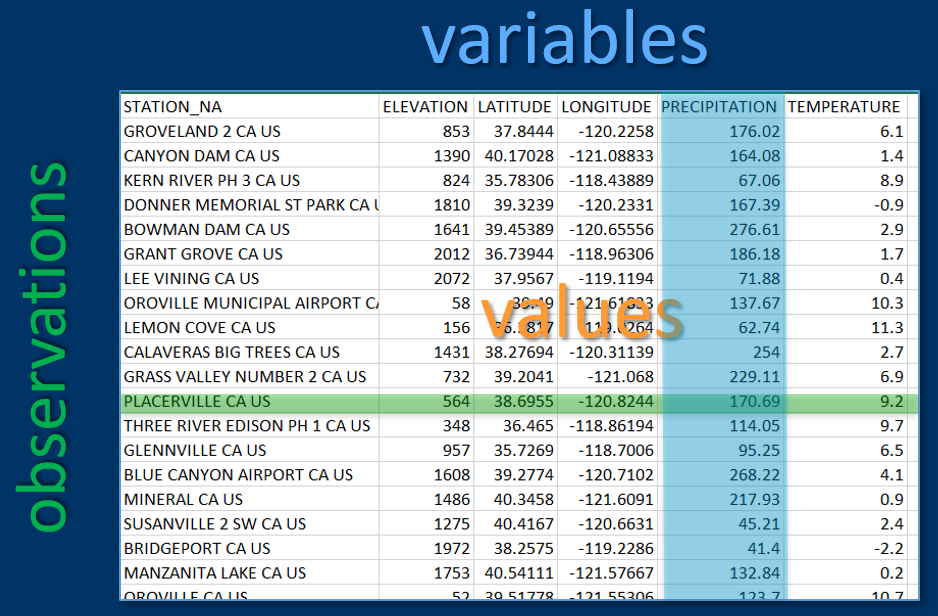FIGURE 2.1: Variables, observations and values in rectangular data

library(igisci)
sierraFeb
## # A tibble: 82 × 7
##    STATION_NAME                   COUNTY ELEVA…¹ LATIT…² LONGI…³ PRECI…⁴ TEMPE…⁵
##    <chr>                          <chr>    <dbl>   <dbl>   <dbl>   <dbl>   <dbl>
##  1 GROVELAND 2, CA US             Tuolu…   853.     37.8   -120.   176.      6.1
##  2 CANYON DAM, CA US              Plumas  1390.     40.2   -121.   164.      1.4
##  3 KERN RIVER PH 3, CA US         Kern     824.     35.8   -118.    67.1     8.9
##  4 DONNER MEMORIAL ST PARK, CA US Nevada  1810.     39.3   -120.   167.     -0.9
##  5 BOWMAN DAM, CA US              Nevada  1641.     39.5   -121.   277.      2.9
##  6 BRUSH CREEK RANGER STATION, C… Butte   1085.     39.7   -121.   296.     NA
##  7 GRANT GROVE, CA US             Tulare  2012.     36.7   -119.   186.      1.7
##  8 LEE VINING, CA US              Mono    2072.     38.0   -119.    71.9     0.4
##  9 OROVILLE MUNICIPAL AIRPORT, C… Butte     57.9    39.5   -122.   138.     10.3
## 10 LEMON COVE, CA US              Tulare   156.     36.4   -119.    62.7    11.3
## # … with 72 more rows, and abbreviated variable names ¹​ELEVATION, ²​LATITUDE,
## #   ³​LONGITUDE, ⁴​PRECIPITATION, ⁵​TEMPERATURE

## 2.6 Data Structures in R

We’ve already started using the most common data structures – scalars and vectors – but haven’t really talked about vectors yet, so we’ll start there.

### 2.6.1 Vectors

A vector is an ordered collection of numbers, strings, vectors, data frames, etc. What we mostly refer to simply as vectors are formally called atomic vectors which requires that they be homogeneous sets of whatever type we’re referring to, such as a vector of numbers,or a vector of strings, or a vector of dates/times.

You can create a simple vector with the c() function:

lats <- c(37.5,47.4,29.4,33.4)
lats
##  37.5 47.4 29.4 33.4
states <- c("VA", "WA", "TX", "AZ")
states
##  "VA" "WA" "TX" "AZ"
zips <- c(23173, 98801, 78006, 85001)
zips
##  23173 98801 78006 85001

The class of a vector is the type of data it holds

temp <- c(10.7, 9.7, 7.7, 9.2, 7.3, 6.7)
class(temp)
##  "numeric"

Let’s also introduce the handy str() function which in one step gives you a view of the class of an item and its content, so its structure. We’ll often use it in this book when we want to tell the reader what a data object contains, instead of listing a vector and its class separately, so instead of …

temp
##  10.7  9.7  7.7  9.2  7.3  6.7
class(temp)
##  "numeric"

… we’ll just use str():

str(temp)
##  num [1:6] 10.7 9.7 7.7 9.2 7.3 6.7

Vectors can only have one data class, and if mixed with character types, numeric elements will become character:

mixed <- c(1, "fred", 7)
str(mixed)
##  chr [1:3] "1" "fred" "7"
mixed   # gets a subset, example of coercion
##  "7"

#### 2.6.1.1 NA

Data science requires dealing with missing data by storing some sort of null value, called various things:

• null
• nodata
• NA “not available” or “not applicable”
as.numeric(c("1","Fred","5")) # note NA introduced by coercion
##   1 NA  5

We often want to ignore NA in statistical summaries. Where normally the summary statistic can only return NA

mean(as.numeric(c("1", "Fred", "5")))
##  NA

… with na.rm=T you can still get the result for all actual data:

mean(as.numeric(c("1", "Fred", "5")), na.rm=T)
##  3

Don’t confuse with nan (“not a number”) which is used for things like imaginary numbers (explore the help for more on this)

is.nan(NA)
##  FALSE
is.na(as.numeric(''))
##  TRUE
is.nan(as.numeric(''))
##  FALSE
i <- sqrt(-1)
is.na(i) # interestingly nan is also na
##  TRUE
is.nan(i)
##  TRUE

#### 2.6.1.2 Creating a vector from a sequence

We often need sequences of values, and there are a few ways of creating them. The following 3 examples are equivalent:

seq(1,10)
1:10
c(1,2,3,4,5,6,7,8,9,10)

The seq() function has special uses like using a step parameter:

seq(2,10,2)
##   2  4  6  8 10

#### 2.6.1.3 Vectorization and vector arithmetic

Arithmetic on vectors operates element-wise, a process called vectorization.

elev <- c(52,394,510,564,725,848,1042,1225,1486,1775,1899,2551)
elevft <- elev / 0.3048
elevft
##    170.6037 1292.6509 1673.2283 1850.3937 2378.6089 2782.1522 3418.6352
##   4019.0289 4875.3281 5823.4908 6230.3150 8369.4226

Another example, with 2 vectors:

temp03 <- c(13.1,11.4,9.4,10.9,8.9,8.4,6.7,7.6,2.8,1.6,1.2,-2.1)
temp02 <- c(10.7,9.7,7.7,9.2,7.3,6.7,4.0,5.0,0.9,-1.1,-0.8,-4.4)
tempdiff <- temp03 - temp02
tempdiff
##   2.4 1.7 1.7 1.7 1.6 1.7 2.7 2.6 1.9 2.7 2.0 2.3

#### 2.6.1.4 Plotting vectors

Vectors of Feb temperature, elevation and latitude at stations in the Sierra:

temp <- c(10.7,  9.7,  7.7,  9.2,  7.3,  6.7,  4.0,  5.0,  0.9, -1.1, -0.8,-4.4)
elev <-   c(52,  394,  510,  564,  725,  848, 1042, 1225, 1486, 1775, 1899, 2551)
lat <- c(39.52,38.91,37.97,38.70,39.09,39.25,39.94,37.75,40.35,39.33,39.17,38.21)

Plot individually by index vs a scatterplot

We’ll use the plot() function to visualize what’s in a vector. The plot() function will create an output based upon its best guess of what you’re wanting to see, and will depend on the nature of the data you provide it. We’ll be looking at a lot of ways to visualize data soon, but it’s often useful to just see what plot() gives you. In this case it just makes a bivariate plot where the x dimension is the sequential index of the vector from 1 through the length of the vector, and the values are in the y dimension. For comparison is a scatterplot with elevation on the x axis (Figure 2.2).

plot(temp)
plot(elev,temp)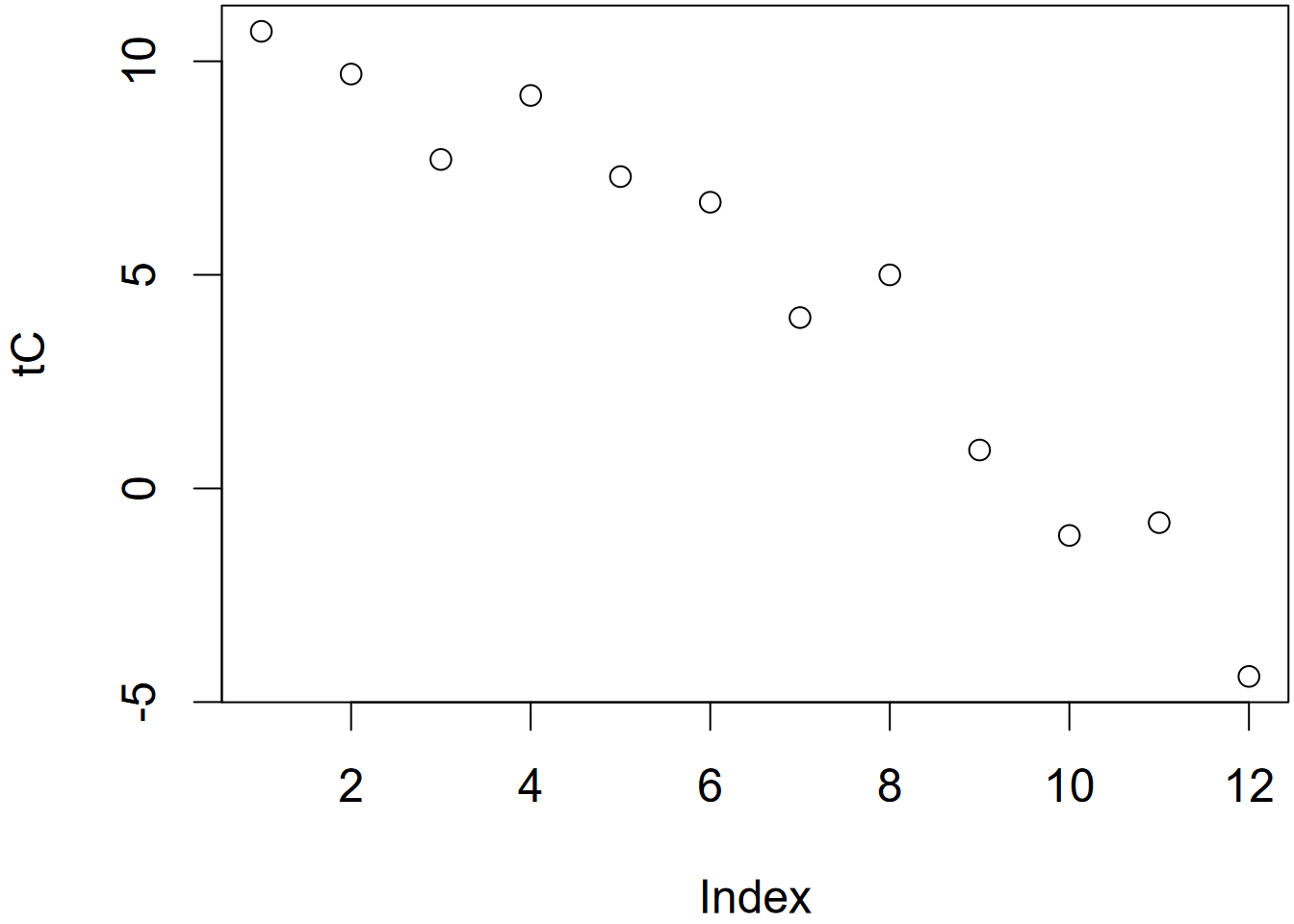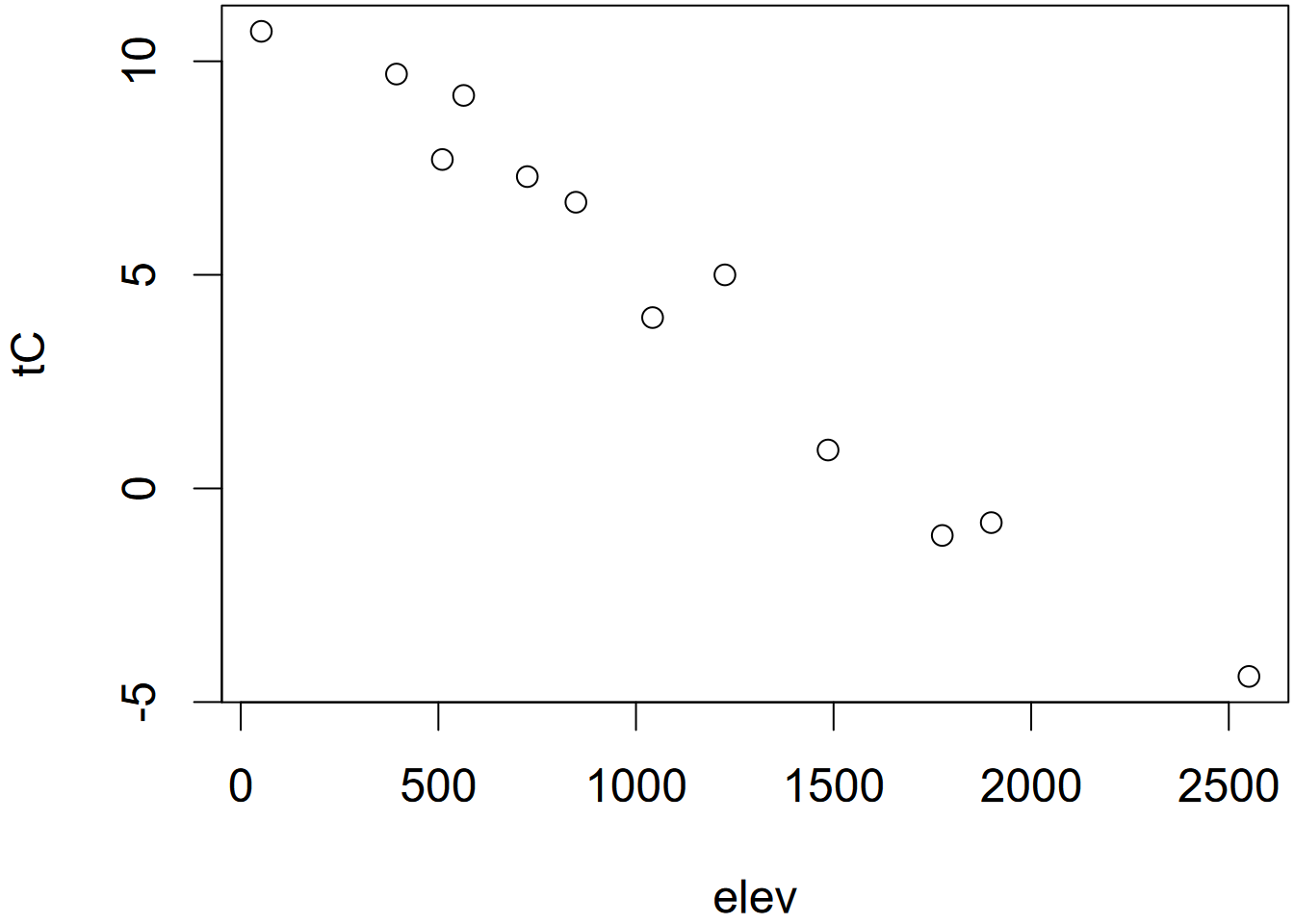FIGURE 2.2: Temperature plotted by index (left) and elevation (right)

#### 2.6.1.5 Named indices

Vectors themselves have names (like elev, temp, and lat above), but individual indices can also be named.

fips <- c(16, 30, 56)
str(fips)
##  num [1:3] 16 30 56
fips <- c(idaho = 16, montana = 30, wyoming = 56)
str(fips)
##  Named num [1:3] 16 30 56
##  - attr(*, "names")= chr [1:3] "idaho" "montana" "wyoming"

The reason we might do this is so you can refer to observations by name instead of index, maybe to filter observations based on criteria where the name will be useful. The following are equivalent:

fips
## montana
##      30
fips["montana"]
## montana
##      30

The names() function can be used to display a character vector of names, or assign names from a character vector:

names(fips) # returns a character vector of names
##  "idaho"   "montana" "wyoming"
names(fips) <- c("Idaho","Montana","Wyoming")
names(fips)
##  "Idaho"   "Montana" "Wyoming"

### 2.6.2 Lists

Lists can be heterogeneous, with multiple class types. Lists are actually used a lot in R, and are created by many operations, but they can be confusing to get used to especially when it’s unclear what we’ll be using them for. We’ll avoid them for a while, and look into specific examples as we need them.

### 2.6.3 Matrices

Vectors are commonly used as a column in a matrix (or as we’ll see, a data frame), like a variable

temp <- c(10.7,  9.7,  7.7,  9.2,  7.3,  6.7,  4.0,  5.0,  0.9, -1.1, -0.8,-4.4)
elev <-   c(52,  394,  510,  564,  725,  848, 1042, 1225, 1486, 1775, 1899, 2551)
lat <- c(39.52,38.91,37.97,38.70,39.09,39.25,39.94,37.75,40.35,39.33,39.17,38.21)

Building a matrix from vectors as columns

sierradata <- cbind(temp, elev, lat)
class(sierradata)
##  "matrix" "array"
str(sierradata)
##  num [1:12, 1:3] 10.7 9.7 7.7 9.2 7.3 6.7 4 5 0.9 -1.1 ...
##  - attr(*, "dimnames")=List of 2
##   ..$: NULL ## ..$ : chr [1:3] "temp" "elev" "lat"
sierradata
##       temp elev   lat
##  [1,] 10.7   52 39.52
##  [2,]  9.7  394 38.91
##  [3,]  7.7  510 37.97
##  [4,]  9.2  564 38.70
##  [5,]  7.3  725 39.09
##  [6,]  6.7  848 39.25
##  [7,]  4.0 1042 39.94
##  [8,]  5.0 1225 37.75
##  [9,]  0.9 1486 40.35
## [10,] -1.1 1775 39.33
## [11,] -0.8 1899 39.17
## [12,] -4.4 2551 38.21

#### 2.6.3.1 Dimensions for arrays and matrices

Note: a matrix is just a 2D array. Arrays have 1, 3, or more dimensions.

dim(sierradata)
##  12  3

It’s also important to remember that a matrix or an array is a vector with dimensions, and we can change those dimensions in various ways as long as they work for the length of the vector.

a <- 1:12
dim(a) <- c(3, 4)   # matrix
class(a)
##  "matrix" "array"
dim(a) <- c(2,3,2)  # 3D array
class(a)
##  "array"
dim(a) <- 12        # 1D array
class(a)
##  "array"
b <- matrix(1:12, ncol=1)  # 1 column matrix is allowed

We just saw that we can change the dimensions of an existing matrix or array. But what if the matrix has names for its columns? I wasn’t sure so following my basic philosophy of empirical programming I just tried it:

dim(sierradata) <- c(3,12)
sierradata
##      [,1] [,2] [,3] [,4] [,5] [,6] [,7] [,8]  [,9] [,10] [,11] [,12]
## [1,] 10.7  9.2  4.0 -1.1   52  564 1042 1775 39.52 38.70 39.94 39.33
## [2,]  9.7  7.3  5.0 -0.8  394  725 1225 1899 38.91 39.09 37.75 39.17
## [3,]  7.7  6.7  0.9 -4.4  510  848 1486 2551 37.97 39.25 40.35 38.21

So the answer is that it gets rid of the column names, and we can also see that redimensioning changes a lot more about how the data appears (though dim(sierradata) <- c(12,3) will return it to its original structure, but without column names). It’s actually a little odd that matrices can have column names, because that really just makes them seem like data frames, so let’s look at those next. Let’s consider a situation where we want to create a rectangular data set from some data for a set of states:

abb <- c("CO","WY","UT")
area <- c(269837, 253600, 84899)
pop <- c(5758736, 578759, 3205958)

We can use cbind to create a matrix out of them, just like we did with the sierradata above

cbind(abb,area,pop)
##      abb  area     pop
## [1,] "CO" "269837" "5758736"
## [2,] "WY" "253600" "578759"
## [3,] "UT" "84899"  "3205958"

But notice what it did – area and pop were converted to character type. This reminds us that matrices are still atomic vectors – all of the same class. So to comply with this, the numbers were converted to character strings, since you can’t convert character strings to numbers.

This isn’t very satisfactory as a data object, so we’ll need to use a data frame, which is not a vector, though its individual column variables are vectors.

### 2.6.4 Data frames

A data frame is a database with variables in columns and rows of observations. They’re kind of like a spreadsheet with rules (like the first row is field names) or a matrix that can have variables of unique types. Data frames will be very important for data analysis and GIS.

Before we get started, we’re going to use the palmerpenguins data set (Figure 2.3), so you’ll need to install it if you haven’t yet, and I’d encourage you to learn more about it at https://allisonhorst.github.io/palmerpenguins/articles/intro.html(Horst, Hill, and Gorman (2020)). It will be useful for a variety of demonstrations using numerical morphometric variables (Figure 2.4) as well as a couple of categorical factors (species and island).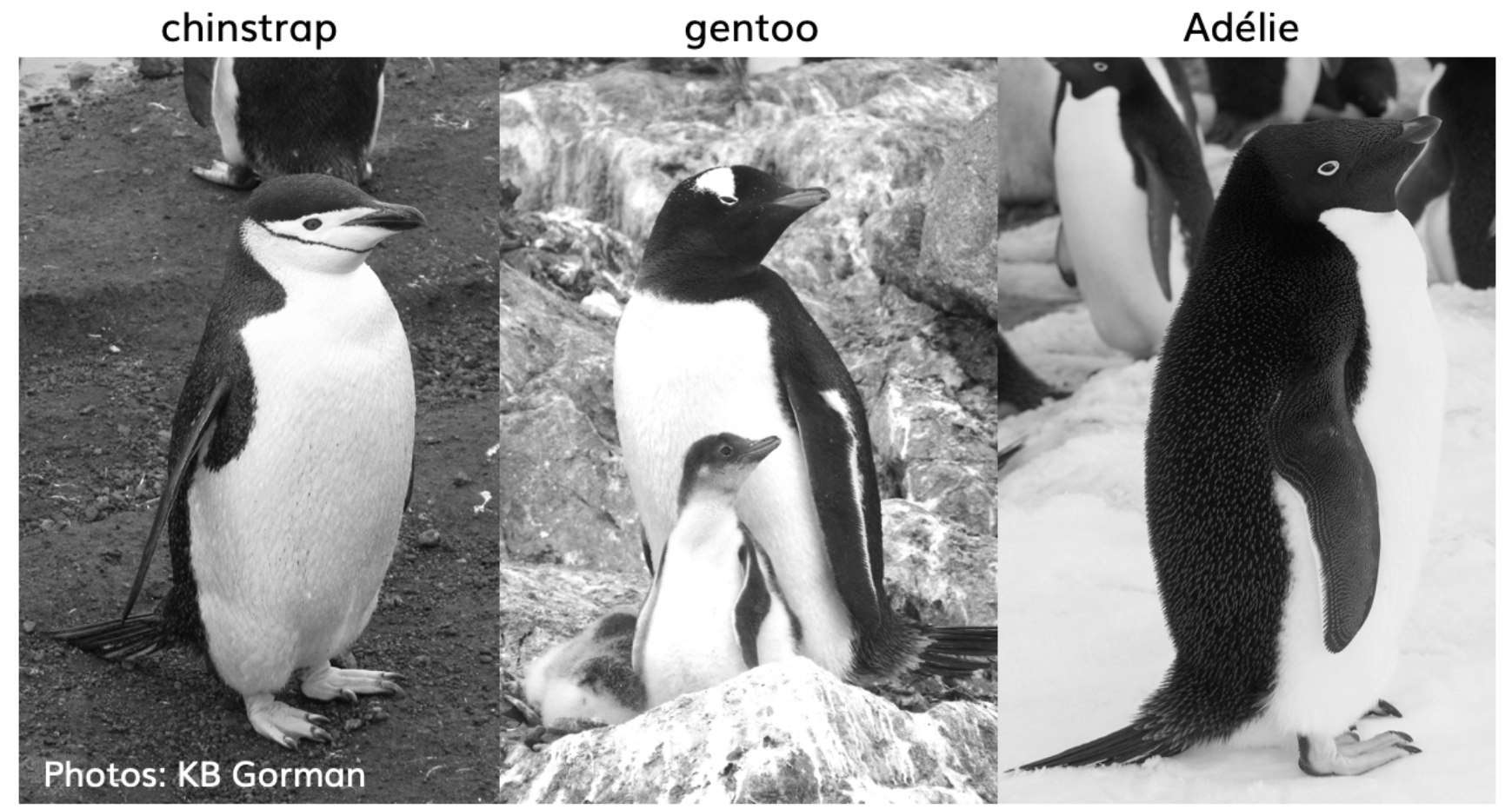FIGURE 2.3: The three penguin species in palmerpenguins. Photos by KB Gorman. Used with permission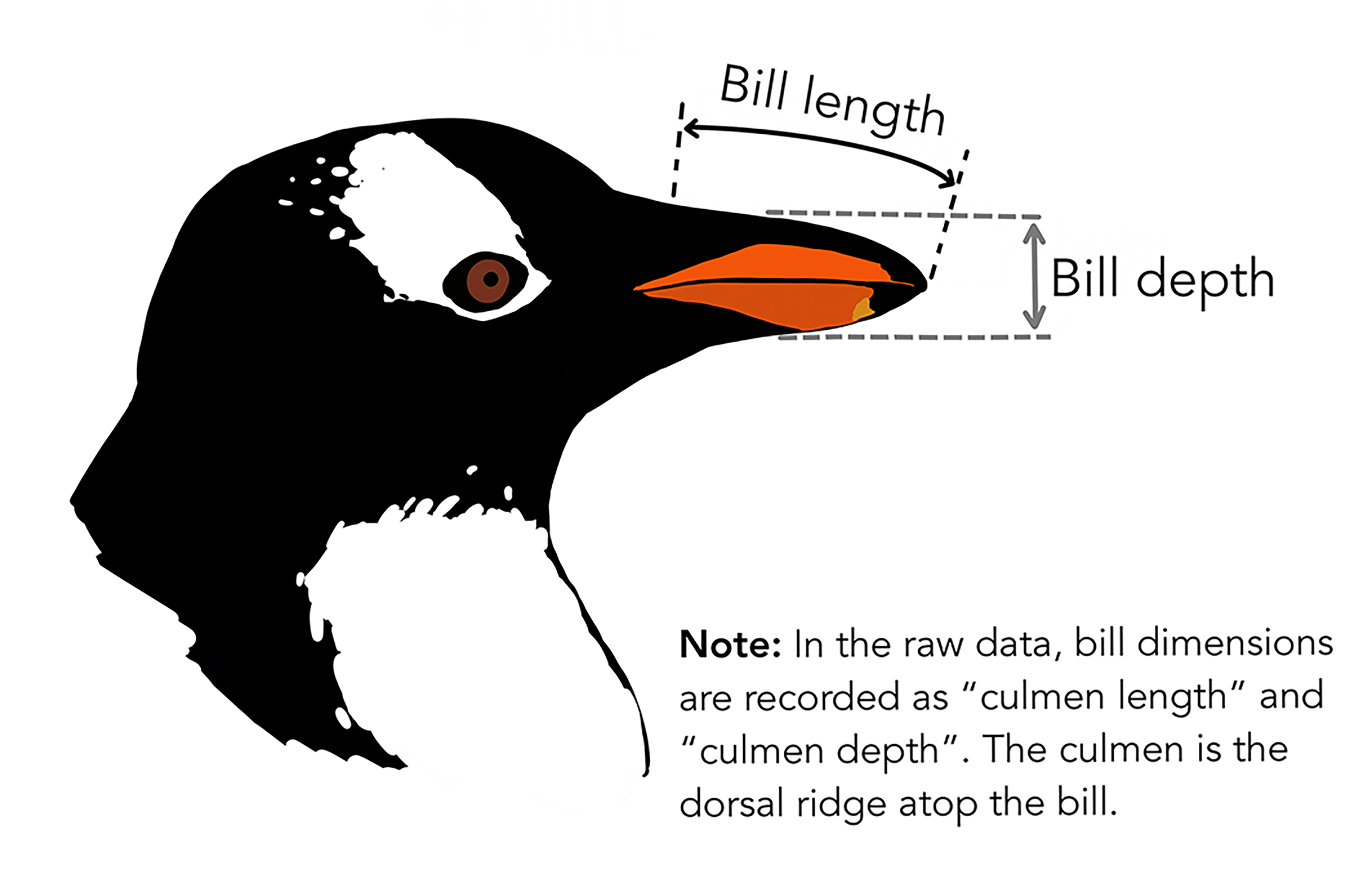FIGURE 2.4: Diagram of penguin head with indication of bill length and bill depth (from Horst, Hill, and Gorman (2020), used with permission)

We’ll use a couple of alternative table display methods, first a simple one…

library(palmerpenguins)
penguins
## # A tibble: 344 × 8
##    species island    bill_length_mm bill_depth_mm flipper_…¹ body_…² sex    year
##    <fct>   <fct>              <dbl>         <dbl>      <int>   <int> <fct> <int>
##  1 Adelie  Torgersen           39.1          18.7        181    3750 male   2007
##  2 Adelie  Torgersen           39.5          17.4        186    3800 fema…  2007
##  3 Adelie  Torgersen           40.3          18          195    3250 fema…  2007
##  4 Adelie  Torgersen           NA            NA           NA      NA <NA>   2007
##  5 Adelie  Torgersen           36.7          19.3        193    3450 fema…  2007
##  6 Adelie  Torgersen           39.3          20.6        190    3650 male   2007
##  7 Adelie  Torgersen           38.9          17.8        181    3625 fema…  2007
##  8 Adelie  Torgersen           39.2          19.6        195    4675 male   2007
##  9 Adelie  Torgersen           34.1          18.1        193    3475 <NA>   2007
## 10 Adelie  Torgersen           42            20.2        190    4250 <NA>   2007
## # … with 334 more rows, and abbreviated variable names ¹​flipper_length_mm,
## #   ²​body_mass_g

… then a nicer table display using DT::datatable, with a bit of improvement using an option.

DT::datatable(penguins, options=list(scrollX=T))

#### 2.6.4.1 Creating a data frame out of a matrix

There are many functions that start with as. that convert things to a desired type. We’ll use as.data.frame() to create a data frame out of a matrix, the same sierradata we created earlier but we’ll build it again so it’ll have variable names, and use yet another table display method from the knitr package (which also has a lot of options you might want to explore) which works well for both the html and pdf versions of this book, and creates numbered table headings, so I’ll use it a lot (Table 2.1).

temp <- c(10.7,  9.7,  7.7,  9.2,  7.3,  6.7,  4.0,  5.0,  0.9, -1.1, -0.8,-4.4)
elev <-   c(52,  394,  510,  564,  725,  848, 1042, 1225, 1486, 1775, 1899, 2551)
lat <- c(39.52,38.91,37.97,38.70,39.09,39.25,39.94,37.75,40.35,39.33,39.17,38.21)
knitr::kable(mydata,
caption = 'Temperatures (Feb), elevations, and latitudes of 12 Sierra stations')
TABLE 2.1: Temperatures (Feb), elevations, and latitudes of 12 Sierra stations
temp elev lat
10.7 52 39.52
9.7 394 38.91
7.7 510 37.97
9.2 564 38.70
7.3 725 39.09
6.7 848 39.25
4.0 1042 39.94
5.0 1225 37.75
0.9 1486 40.35
-1.1 1775 39.33
-0.8 1899 39.17
-4.4 2551 38.21

Then to plot the two variables that are now part of the data frame, we’ll need to make vectors out of them again using the $ accessor (Figure 2.5). plot(mydata$elev, mydata\$temp)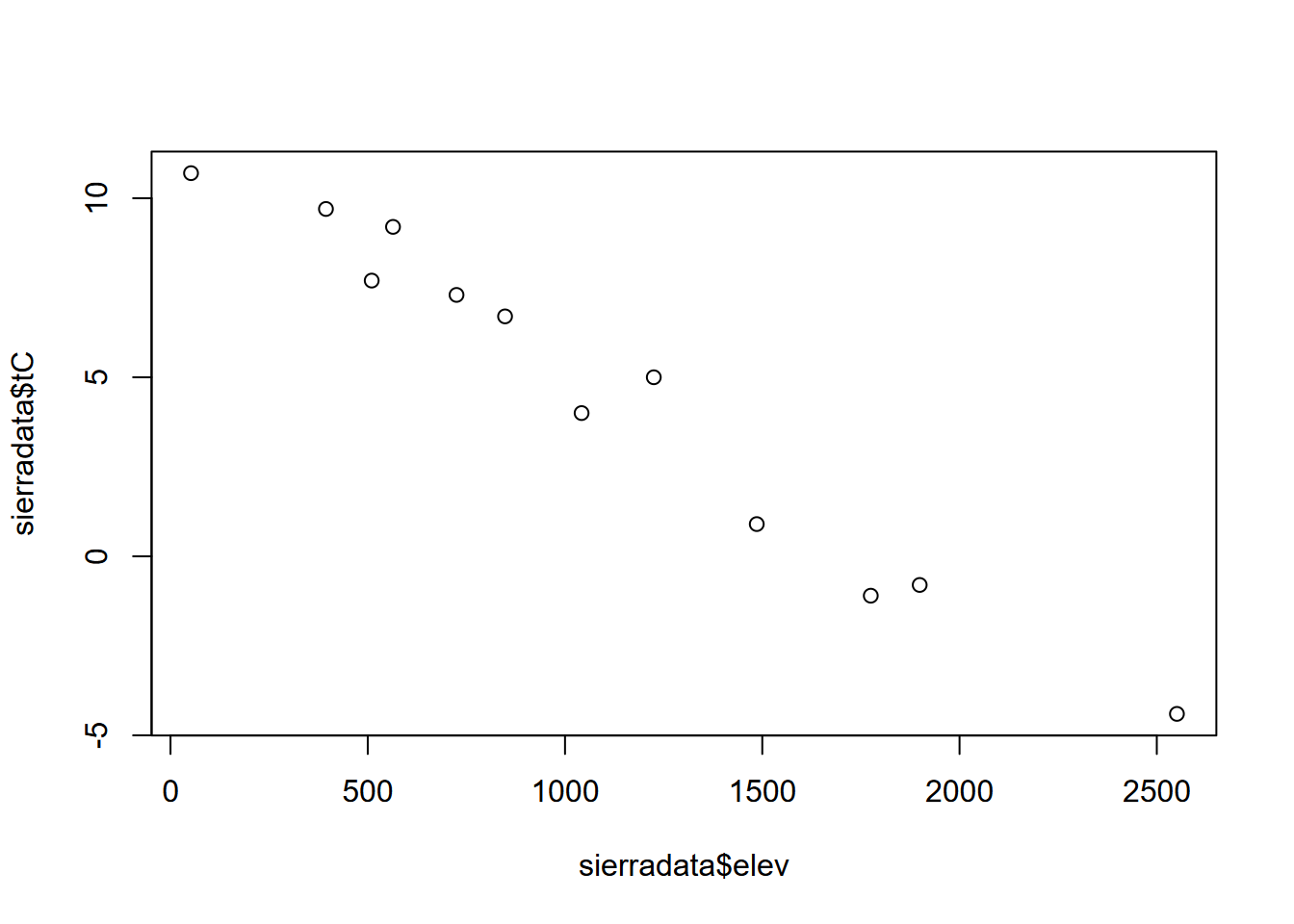FIGURE 2.5: Temperature~Elevation

#### 2.6.4.2 Read a data frame from a CSV

We’ll be looking at this more in the next chapter, but a common need is to read data from a spreadsheet stored in the CSV format. Normally, you’d have that stored with your project and can just specify the file name, but we’ll access CSVs from the igisci package. Since you have this installed, it will already be on your computer, but not in your project folder. The path to it can be derived using the system.file() function.

Reading a csv in readr (part of the tidyverse that we’ll be looking at in the next chapter) is done with read_csv(). We’ll use the DT::datatable for this because it lets you interactively scroll across the many variables, and this table is included as Figure 2.6.

library(readr)
csvPath <- system.file("extdata","TRI/TRI_2017_CA.csv", package="igisci")
TRI2017 <- read_csv(csvPath)
DT::datatable(TRI2017, options=list(scrollX=T))